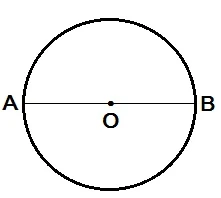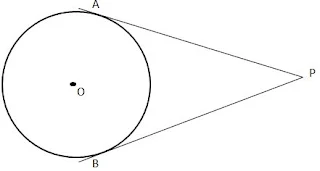#### Study Materials and Revision Notes for Ch 10 Circle Class 10th Maths

Circle

Circle: A circle is a locus of a point which moves in such a way that the distance from that point is always fixed.

Radius: The constant distance from the centre to the circumference of the circle.

Secant: A line which intersect the circle at two different points.

Chord: Any line segment joining the two points on the circumference of the circle.

Diameter: The longest distance between the two points on the circumference of the circle. It is the longest chord.Here, AO is the radius of the circle and AB is the diameter of the circle.

Tangent

Tangent: A line which touches the circle exactly at one point.

• A line which touches the circle exactly at one point.

• The tangent at any point on a circle is perpendicular to the radius through the point of contact.• In the above diagram l is tangent to the circle at point P. O is center, Then OP is perpendicular to l.
OP ⊥ l.

Tangent drawn from an external point to a circle
Length of the tangent: The length of the segment of the tangent from an external point P to the point of contact with the circle is called the length of the tangent from the point P to the circle.

Theorem: The lengths of tangents drawn from an external point to a circle are equal.Two tangents are drawn from a point P, PA and PB.
PA=PB.